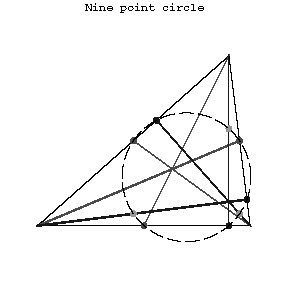# Nine-point circle

(diff) ← Older revision | Latest revision (diff) | Newer revision → (diff)
A circle whose periphery contains the midpoints of the sides of a triangle, the bases of its altitudes, and the midpoints of the segment connecting the orthocentre of the triangle with the vertices. Its radius is equal to one-half of the radius of the circle circumscribed about the triangle. The nine-point circle of a triangle is tangent to the circle inscribed in it and to the three escribed circles. Letbe the orthocentre of a non-equilateral triangle, letbe the centre of gravity, let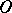be the centre of the circumscribed circle and letbe the centre of the nine-point circle. The pointsthen lie on a straight line (Euler's line),being the midpoint of the segment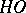, and the pair of points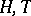harmonically subdivides the pair of points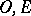.